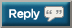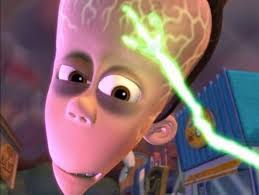# Thread: is it justified to be racist / prejudice if..

1. ## is it justified to be racist / prejudice if..

that group of people destroys the ****ing world?2. Confused... Has the world been destroyed?3. Isn’t that like saying should EVERY white person be blamed for slavery of blacks in the US? Obviously you had nothing to do with it, true?4. Not sure if you know where you're posting, but this is the misc section, where all homophobic, racial slurs has been deemed accepted in this society.5. that group of people destroys the ****ing world?
yo can you help me understand something before i smash my ****ing computer

2. (a) Use the existence of the extended Euclidean algorithm to prove that if n is greater than 1, and a ∈ ℤn such that
gcd(n, a) = 1, then a has a multiplicative inverse mod n.
(b) Compute the determine the multiplicative inverse of 400 in ℤ1001.6. yo can you help me understand something before i smash my ****ing computer

2. (a) Use the existence of the extended Euclidean algorithm to prove that if n is greater than 1, and a ∈ ℤn such that
gcd(n, a) = 1, then a has a multiplicative inverse mod n.
(b) Compute the determine the multiplicative inverse of 400 in ℤ1001.
a) The extended Euclidean algorithm computes the pair of minimal coefficients for Bezout's identity. These coefficients will (in this case) will be less than n in absolute value (in general they will be less than n/gcd(a,n) in absolute value). There are two minimal pairs, and it allows us to make a choice of sign. Remember that **** because I will reference it in a second bro.

Suppose that gcd(n,a) = 1.

Then by the extended Euclidean euclidean algorithm we have the minimal integers (as described above) x,y such that

ax + ny = gcd(a,n) = 1

So

ax + ny = 1

So if we rearrange this,

ax = n(-y) + 1

If a = 1, then its multiplicative inverse is itself and we are done. If a > 1, then remember all that **** I said about the minimality of coefficients. Pick the minimal pair where y is negative and x is positive, let's give -y a name, say k = -y > 0. Recall that x is less than n in absolute value. Hence 0 < x < n, so x is in Z mod n (!).

Now we have

ax = nk + 1

So... we're done. We multiplied a by x (another element of Z mod n) and got a multiple of n, plus 1. So ax = 1 mod n. The multiplicative inverse of a is x: the minimal positive coefficient of Bezout's identity on a that is computed by the extended Euclidean algorithm. Use that sentence I just said to do (b).Also this is why Z mod p always forms a field.7. Isn’t that like saying should EVERY white person be blamed for slavery of blacks in the US? Obviously you had nothing to do with it, true?
to be fair i know a lot of black guys that hold resentment towards whites because of this8. Confused... Has the world been destroyed?
You must be pretty confused in general if you don't know how if statements work.9. Europeans whites came to the united states with their diseases and wiped out +millions of native americans across the continent10. Only if we’re talking about the Chinamen

Fukk those guys11. to be fair i know a lot of black guys that hold resentment towards whites because of this
200 year old black men?#### Posting Permissions

• You may not post new threads
• You may not post replies
• You may not post attachments
• You may not edit your posts